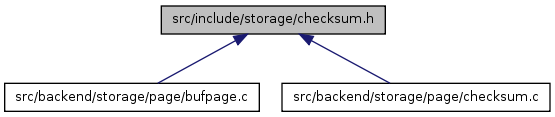# checksum.h File Reference

This graph shows which files directly or indirectly include this file:Go to the source code of this file.

## Functions

uint32 checksum_block (char *data, uint32 size)

## Function Documentation

 uint32 checksum_block ( char * data, uint32 size )

Definition at line 132 of file checksum.c.

References Assert, CHECKSUM_COMP, checksumBaseOffsets, i, and N_SUMS.

Referenced by PageCalcChecksum16().

```{
uint32 sums[N_SUMS];
uint32 (*dataArr)[N_SUMS] = (uint32 (*)[N_SUMS]) data;
uint32 result = 0;
int i, j;

/* ensure that the size is compatible with the algorithm */
Assert((size % (sizeof(uint32)*N_SUMS)) == 0);

/* initialize partial checksums to their corresponding offsets */
memcpy(sums, checksumBaseOffsets, sizeof(checksumBaseOffsets));

/* main checksum calculation */
for (i = 0; i < size/sizeof(uint32)/N_SUMS; i++)
for (j = 0; j < N_SUMS; j++)
CHECKSUM_COMP(sums[j], dataArr[i][j]);

/* finally add in two rounds of zeroes for additional mixing */
for (i = 0; i < 2; i++)
for (j = 0; j < N_SUMS; j++)
CHECKSUM_COMP(sums[j], 0);

/* xor fold partial checksums together */
for (i = 0; i < N_SUMS; i++)
result ^= sums[i];

return result;
}
```